# Introduction to Dex

Dex is a functional, statically typed language for array processing. There are many tools for array processing, from high-level libraries like NumPy and MATLAB to low-level languages like CUDA. Dex is a new approach for high-level array processing that aims for the clarity of high-level libraries while allowing for more granular expressivity. In particular, Dex does not force you to rewrite all operations in terms of batched tensor interactions, but allows for a range of interactions. Put more simply, when learning MATLAB students are told repeatedly to "avoid for loops". Dex gives for loops back.

## Table comprehensions

Let us begin with the most useful component of Dex, the for builder. The best analogy for this construct is list comprehensions in Python. For instance, in Python, we might write a list comprehension like:

x = [[1.0 for j in range(width)] for i in range(height)]

In Dex, this construct would be written as:

Height = Fin 3
Width = Fin 8
x = for i:Height. for j:Width. 1.0

Once we have a variable, we can print it.

x
[ [1., 1., 1., 1., 1., 1., 1., 1.] , [1., 1., 1., 1., 1., 1., 1., 1.] , [1., 1., 1., 1., 1., 1., 1., 1.] ]

More interestingly, we can also see its type with :t. This type tells us that x is a two-dimensional table, whose first dimension has type Height and second dimension has type Width.

:t x
((Fin 3) => (Fin 8) => Float32)

Here Fin stands for finite represents the type of range from 0 to the value given minus one. The : tells us the type of the enumeration variable.

We can also display it as html. To do this we include the plot library. Right now our table is not so interesting :)

import png
import plot
:html matshow xOnce we have an table, we can use it in new comprehensions. For example, let's try to add 5 to each table element. In Python, one might write this as:

x5 = [[x[i][j] + 5.0 for j in range(width)] for i in range(height)]

Dex can do something similar. The main superficial difference is the table indexing syntax, which uses table.i instead of square brackets for subscripting.

:t for i:Height. for j:Width. x.i.j + 5.0
((Fin 3) => (Fin 8) => Float32)

However, we can make this expression nicer. Because x has a known table type and i and j index into that type, Dex can infer the range of the loop. That means that we can safely remove the explicit Fin type annotations and get the same result.

:t for i. for j. x.i.j + 5.0
((Fin 3) => (Fin 8) => Float32)

Dex also lets you reduce this expression to include multiple variables in the same for.

:t for i j. x.i.j + 5.0
((Fin 3) => (Fin 8) => Float32)

Standard functions can be applied as well. Here we take the mean over each column:

:t for i. mean x.i
((Fin 3) => Float32)

This style of using for to construct type-inferred tables is central to what makes Dex powerful. Tables do not only need to have Fin types.

Let's consider another example. This one produces a list of ones in Python.

y = [1.0 for j in range(width) for i in range(height)]

The analogous table construct in Dex is written in the following form. It produces a one-dimensional table of Height x Width elements. Here & indicates a tuple constructor.

y = for (i, j) : (Height & Width) . 1.0

As before, we can implement "adding 5" to this table using a for constructor, enumerating over each of its elements:

y5 = for i. y.i + 5.0

And we can apply table functions to the table:

mean y
1.

But things start to get interesting when we consider the type of the table. Unlike the Python example, which produces a flat list (or other examples like NumPy arrays), the Dex table maintains the index type of its construction. In particular, the type of the table remembers the original ranges.

:t y
((Fin 3 & Fin 8) => Float32)

## Typed indexing

The use of typed indices lets you do really neat things, but let's consider how it works. Critically, one cannot simply index an table with an integer.

r = x.(2 : Int)
Type error: Expected: (Fin 3) Actual: Int32 r = x.(2 : Int) ^^

Instead, it is necessary to cast the integer into the index type of the current shape. This type annotation is done with the @ operator.

:t x
((Fin 3) => (Fin 8) => Float32)
row = x.(2 @ Height)
:t row
((Fin 8) => Float32)
:t row.(5 @ Width)
Float32

If you are feeling lazy and sure of yourself, you can also let Dex infer the type for you. This is also how for works in the examples above that did not provide and explicit type annotation.

:t row.(5 @ _)
Float32

If it helps, you can think of table indexing as function application: a.i applies table a with index i just like how f x applies function f with argument x.

Another consequence is that you cannot use indices as integers. It is necessary to explicitly annotate index types with ordinal. This is because finite sets i.e. Fin are not closed under addition.

:t for i:Height. for j:Width. i + j
Type error: Expected: (Fin 3) Actual: (Fin 8) :t for i:Height. for j:Width. i + j ^
:t for i:Height. for j:Width. (ordinal i) + (ordinal j)
((Fin 3) => (Fin 8) => Nat)

If we want to convert these values to floats, we do it manually with the IToF function. We can use this to make an image gradient.

gradient = for i:Height. for j:Width. n_to_f ((ordinal i) + (ordinal j))As we have seen, indices are not limited to only integers. Many different Dex types are valid index types. For example, we declared table y as having a pair of integers as its index type (a & b means tuple type), so indexing into y requires creating a tuple value.

:t y
((Fin 3 & Fin 8) => Float32)
:t y.(2 @ Height, 5 @ Width)
Float32

Tuple indices also provide an ordinal value.

for pair:(Fin 2 & Fin 3). ordinal pair
[0, 1, 2, 3, 4, 5]@(Fin 2 & Fin 3)

Many algorithms in Dex come down to being able to pack and unpack these indices. For example, we have seen that it is easy to sum over one dimension of a 2D table. However, if we have a 1D table indexed by a pair, we can easily turn it into a 2D table using two for constructors.

:t for i. for j. y.(i, j)
((Fin 3) => (Fin 8) => Float32)

Again, we rely on type inference in order to avoid explicitly spelling the ranges.

## Defining functions over tables

One use case of packing and unpacking table indices is that it allows us to change the order of the axes. This is useful for applying functions on tables.

For instance, we saw the mean function above which sums over the first axis of an table. We can apply mean to y to produce the mean average over 24 elements:

:t y
((Fin 3 & Fin 8) => Float32)
:t mean y
Float32

The mean function works independently of the index type of the table.

Let's see how we can define our own table functions. Defining a function in Dex uses the following syntax.

def add5 (x:Float32) : Float32 = x + 5.0
6.
((Fin 3 & Fin 8) => Float32)

Functions also have types. Note that that function types in Dex use the -> symbol whereas tables use =>.

(Float32 -> Float32)

We can also write functions with type variables over their inputs. For instance, we may want to be able to write a function that applies "adds 5" to tables with any index type. This is possible by declaring an n => Int32 table argument type: this declares the type variable n as the index type of the table argument.

def tableAdd5' {n} (x : n => Float32) : n => Float32 = for i. x.i + 5.0
((Fin 3 & Fin 8) => Float32)

But function types can help you out even more. For instance, since index types are statically known, type checking can ensure that table arguments have valid dimensions. This is "shape safety".

Imagine we have transpose function. We can encode the shape change in the type.

def transFloat {m n} (x : m => n => Float32) : n => m => Float32 = for i. for j. x.j.i

We can even make it more generic by abstracting over the value type.

def trans {m n v} (x : m => n => v) : n => m => v = for i. for j. x.j.i

We can also use this to check for shape errors:

:t x
((Fin 3) => (Fin 8) => Float32)
def tableAdd' {m n} (x : m => n => Float32) (y : m => n => Float32) : m => n => Float32 = for i. for j. x.i.j + y.i.j
((Fin 3) => (Fin 8) => Float32)
Type error: Expected: ((Fin 3) => (Fin 8) => Float32) Actual: ((Fin 8) => (Fin 3) => Float32) :t tableAdd' x (trans x) ^^^^^^^

The type system checked for us that the input tables indeed have the same shape.

## Case Study: Fashion MNist

To run this section, move the following binary files to examples:

wget http://fashion-mnist.s3-website.eu-central-1.amazonaws.com/t10k-images-idx3-ubyte.gz; gunzip t10k-images-idx3-ubyte.gz

wget http://fashion-mnist.s3-website.eu-central-1.amazonaws.com/t10k-labels-idx1-ubyte.gz; gunzip t10k-labels-idx1-ubyte.gz

To make some of these concepts for tangible let us consider a real example using Fashion MNist clothing. For this example we will first read in a batch of images each with a fixed size.

Batch = Fin 5000
IHeight = Fin 28
IWidth = Fin 28
Channels = Fin 3
Class = Fin 10
Image = (IHeight => IWidth => Float)
Full = Fin ((size Batch) * (size IHeight) * (size IWidth))

To do this we will use Dex's IO to load some images from a file. This section uses features outside the scope of the tutorial, so you can ignore it for now.

def pixel (x:Char) : Float32 = r = w8_to_i x i_to_f case r < 0 of True -> 256 + r False -> r
def getIm : Batch => Image = -- File is unsigned bytes offset with 16 starting bytes (AsList _ im) = unsafe_io do read_file "examples/t10k-images-idx3-ubyte" raw = unsafe_cast_table Full (for i:Full. im.((ordinal i + 16) @ _)) for b: Batch i j. pixel raw.((ordinal (b, i, j)) @ Full)
def getLabel : Batch => Class = -- File is unsigned bytes offset with 8 starting bytes (AsList _ lab) = unsafe_io do read_file "examples/t10k-labels-idx1-ubyte" r = unsafe_cast_table Batch (for i:Batch. lab.((ordinal i + 8) @ _)) for i. (w8_to_n r.i @ Class)
all_ims = getIm
all_labels = getLabel
ims = for i : (Fin 100). all_ims.(ordinal i@_)
im = ims.(0 @ _)
:html matshow im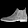:html matshow ims.(1 @ _)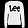This show the mean pixel value aggregation over all images.

:html matshow (sum ims)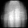This example overplots three different pairs of clothing.

imscolor = for i. for j. for c:Channels. ims.((ordinal c)@_).i.j
:t imscolor
((Fin 28) => (Fin 28) => (Fin 3) => Float32)
:html imshow (imscolor / 255.0)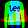This one shows all the images on one channel over the base plot.

imscolor2 = for b. for i. for j. for c:Channels. case ordinal c == 0 of True -> (sum ims).i.j / (n_to_f (size Batch)) False -> ims.b.i.j
:html imseqshow (imscolor2 / 255.0)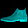This example utilizes the type system to help manipulate the shape of an image. Sum pooling downsamples the image as the max of each pixel in a tile grid pattern. See if you can figure out the other types.

def split {m n o v} [Ix n, Ix o] (x: m=>v) : n=>o=>v = for i j. x.(ordinal (i,j)@_)
def imtile {a b n o p q v} [Ix n, Ix o, Ix p, Ix q] (x: a=>b=>v) : n=>o=>p=>q=>v = for kh kw h w. (split (split x).h.kh).w.kw
im1 : Fin 2 => Fin 2 => Fin 14 => Fin 14 => Float32 = imtile ims.(0@_)
:html matshow (sum (sum im1))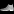im2 : Fin 4 => Fin 4 => Fin 7 => Fin 7 => Float32 = imtile ims.(0@_)
:html matshow (sum (sum im2))## Writing Loops

Dex is a functional language - but when writing mathematical algorithms, it is often convenient to temporarily put aside immutability and write imperative code using mutation.

For example, let's say we want to actually implement the mean function ourselves by accumulating summed values in-place. In Python, implementing this is not directly possible solely via list comprehensions, so we would write a loop.

acc = 0.0

for i in range(len(x)):

  acc = acc + x[i]


return acc / len(x)

In Dex, values are immutable, so we cannot directly perform mutation. But Dex includes algebraic effects, which are a purely-functional way to modeling side-effects like mutation. We can write code that looks like mutation using the State effect, which provides getter and setter functionality (via get and := assignment). Here's what it looks like:

def tableMean {n} (x : n => Float32) : Float32 = -- acc = 0 with_state 0.0 $\acc. -- for i in range(len(x)) for i. -- acc = acc + x[i] acc := (get acc) + x.i -- return acc / len(x) (get acc) / (n_to_f (size n)) tableMean [0.0, 1.0, 0.5] 0.5 So, even though Dex is a functional language, it is possible to write loops that look similar to ones that truly perform mutation. However, there is one line which is quite new and a bit scary. Let us look into that line in a bit more detail. First: $. This symbol is used in Dex just like it is used in Haskell, but if you haven't seen it before, it seems a bit strange. The symbol $ is the function application operator: it basically replaces of expression-grouping parentheses (f x) when it is inconvenient to write them. For example, the following two expressions are identical: :t tableMean (y + y) Float32 :t tableMean$ y + y
Float32

Next: \. This symbol is the lambda sigil in Dex. It is analogous to the lambda keyword in Python, and starts the definition of a function value (i.e. closure). In tableMean above: the lambda takes an argument named acc and returns the body, which is the expression following the . (a for constructor in this case).

:t \ x. x + 10
(Nat -> Nat)
(\ x. x + 10) 20
30

That leaves: withState. This function uses the State effect, enabling us to introduce imperative variables into the computation. withState takes an initial value init and a body function taking a "mutable value" reference (acc here), and returns the body function's result. Here's a simple example:

with_state 10 $\ state. state := 30 state := 10 get state 10 The element returned is the body function's result (10) Finally: this is a good point to talk a bit about some other operators in Dex. In the examples above, we see two types of equal sign operators: = and :=. The first is the let operator that creates an immutable assignment (a "let-binding"). This one is built into the language and can be used anywhere. :t for i:Height. -- Bind a temporary variable temp, as an example. temp = (ordinal i) + 10 for j:Width. temp ((Fin 3) => (Fin 8) => Nat) The other is :=, which is an assignment operator that can only be used when a State effect is available (e.g. inside of a body function passed to withState). ref := x assigns the value x to the mutable reference ref. Reading the value in ref is possible via the get function. or via using the final result returned by withState. ## Interfaces Our tableMean function is pretty neat. It lets us work with tables with any index type and computes the sum. However, tableMean explicitly takes only integer tables (of type n => Float32). :t tableMean ((n:Type) ?-> (_autoq:(Ix n)) ?=> (n => Float32) -> Float32) If we try to apply tableMean to other types for get errors. For example, tableMean does not work when applied to a table of pairs of floats. :t (for (i, j). (x.i.j, x.i.j)) ((Fin 3 & Fin 8) => (Float32 & Float32)) tableMean (for (i, j). (x.i.j, x.i.j)) Type error: Expected: Float32 Actual: (Float32 & Float32) tableMean (for (i, j). (x.i.j, x.i.j)) ^^^^^^^^^^^^ Intuitively, supporting this seems possible. We just need to be able to add and divide pair types. Let's look closer at the exact types of the addition and division operators. :t (+) ((a:Type) ?-> (Add a) ?=> a -> a -> a) :t (/) ((a:Type) ?-> (VSpace a) ?=> a -> Float32 -> a) These function types are a bit complex. (+) maps a -> a -> a with a constraint that a implements the Add' interface. Whereas (/) maps a -> Float32 -> a where a implements the VSpace' interface. If we look in the Prelude, we can see that these interfaces are defined as (This will throw error because it mirrors the prelude, but we are just repeating it here.): interface Add a add : a -> a -> a sub : a -> a -> a zero : a  interface [Add a] VSpace a scaleVec : Float -> a -> a  Interfaces define requirements: the functions needed for a type to implement the interface (via an instance). Here is an Add instance for the float pair type. instance Add (Float32 & Float32) add = \(x1,x2) (y1, y2). (x1 + y1, x2 + y2) zero = (0.0, 0.0) instance Sub (Float32 & Float32) sub = \(x1,x2) (y1, y2). (x1 - y1, x2 - y2) And here is a VSpace instance for the float pair type: instance VSpace (Float32 & Float32) scale_vec = \s (x, y). (x * s, y * s) Once we have these two instance definitions, we can revisit our table sum function using them: def tableMean' {n v} [VSpace v] (x : n => v) : v = with_state zero$ \acc: (Ref _ v). for i. acc := add (get acc) x.i -- Add requirement (get acc) / (n_to_f (size n)) -- VSpace requirement
tableMean' [0.1, 0.5, 0.9]
0.5
tableMean' [(1.0, 0.5), (0.5, 0.8)]
(0.75, 0.65)

The instance values are hardcoded for the float pair type. To be more general, we can and should instead define Add and VSpace instances for generic ' tuple types.

instance {v w} [Add v, Add w] Add (v & w) add = \(x1,x2) (y1, y2). (x1 + y1, x2 + y2) zero = (zero, zero)
instance {v w} [Sub v, Sub w] Sub (v & w) sub = \(x1,x2) (y1, y2). (x1 - y1, x2 - y2)
instance {v w} [VSpace v, VSpace w] VSpace (v & w) scale_vec = \s (x, y). (scale_vec s x, scale_vec s y)

## More Fashion MNist

Now that we have more functions we can revisit some of the Fashion MNist examples.

Function that uses state to produce a histogram:

Pixels = Fin 256
def bincount {a b} [Ix b] (inp : a => b) : b => Nat = with_state zero \acc . for i. v = acc!(inp.i) v := (get v) + 1 get acc

Plot how many times each pixel value occurs in an image:

hist = bincount $for (i,j). (f_to_n (ims.(0 @ _).i.j) @ Pixels) :t hist ((Fin 256) => Nat) :html show_plot$ y_plot (for i. (n_to_f hist.i))

Find nearest images in the dataset:

def imdot {m n} (x : m=>n=>Float32) (y : m=>n=>Float32) : Float32 = sum for (i, j). x.i.j * y.i.j
dist = for b1. for b2. case b1 == b2 of True -> 0.0 False -> -imdot ims.b1 ims.b2
nearest = for i. argmin dist.i
double = for b i j. [ims.b.i.j, ims.(nearest.b).i.j, 0.0]
:html imseqshow double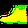## Variable Length Lists

So far all the examples have assumed that we know the exact size of our tables. This is a common assumption in array languages, but it makes some operations surprisingly difficult to do.

For instance, we might want to filter our set of images to only allow for images of 5's. But what is the type of this table?

Dex allows for tables with an unknown and varying length using the List construct. You can think of list as hiding one finite dimension of a table.

:t for i:Height. 0.0
((Fin 3) => Float32)
to_list for i:Height. 0.0
(AsList 3 [0., 0., 0.])
:t to_list for i:Height. 0.0
(List Float32)

Tables of lists can be concatenated down to single lists.

z = concat [to_list [3.0], to_list [1.0, 2.0 ]]
z
(AsList 3 [3., 1., 2.])

And they can be deconstructed to fetch a new table.

(AsList _ temptab) = z
temptab
[3., 1., 2.]

Using this construct we can return to extracting items with label of shoes (label 5) from the image set.

shoes = (5 @ Class)
def findShoes {a b} (x : a=>b) (y : a=>Class) : List b = concat for i. case (y.i == (5 @ _)) of True -> to_list [x.i] False -> to_list []

Note though that the type here does not tell us how many there are. The type system cannot know this. To figure it out we need to unpack the list.

temp = findShoes all_ims all_labels
(AsList nShoes allShoes) = temp
nShoes
485

However we can still utilize the table. For instance if we are summing over the hidden dimension, we never need to know how big it is.

:html matshow (sum allShoes)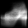## Conclusion

We hope this gives you enough information to start playing with Dex. This is just a start though of the different functionality available in the language. If you are interested in continuing to learn, we recommend you look at the examples in the examples/ directory, check out the prelude in lib/prelude.dx, and file issues on the GitHub repo. We have a welcoming and excited community, and we hope you are interested enough to join us.

Here are some topics to check out in the Prelude:

• Randomness and Keys
• Laziness of For Comprehensions
• Records and Variant Types
• File IO
• Effects Beyond State